Courses

# Complex Number NAT Level - 1

## 10 Questions MCQ Test Topic wise Tests for IIT JAM Physics | Complex Number NAT Level - 1

Description
This mock test of Complex Number NAT Level - 1 for Physics helps you for every Physics entrance exam. This contains 10 Multiple Choice Questions for Physics Complex Number NAT Level - 1 (mcq) to study with solutions a complete question bank. The solved questions answers in this Complex Number NAT Level - 1 quiz give you a good mix of easy questions and tough questions. Physics students definitely take this Complex Number NAT Level - 1 exercise for a better result in the exam. You can find other Complex Number NAT Level - 1 extra questions, long questions & short questions for Physics on EduRev as well by searching above.
*Answer can only contain numeric values
QUESTION: 1

### If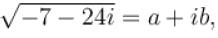then find value of a3 + b3 :

Solution: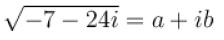by squaring both side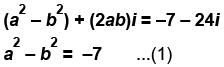2ab = –24 ...(2)
By, (1)2 + (2)2 are get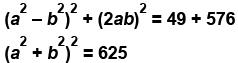a2 + b2 = 25   ...(3)
2a2 = 18
⇒ a = ±3
and   b = ±4
from (2) product of ab  is negative so
a = +3  and  b = –4
or  a = –3  and  b = 4
so a3 + b3 = 27 – 64 = –37
or a3 + b3 = –27 + 64 = 37

*Answer can only contain numeric values
QUESTION: 2

### If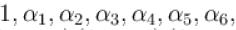are given, 7th root of unity then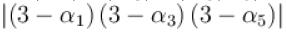is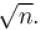Find n.

Solution: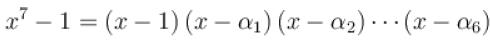put  x = 3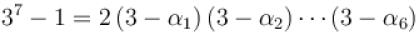but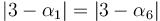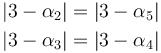So,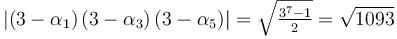*Answer can only contain numeric values
QUESTION: 3

### Sum of common roots of z2006 + z100 + 1 = 0  and  z3 + 2z2 + 2z + 1 = 0 is:

Solution: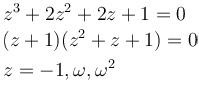Only ω and ω2 satisfy 1st equation
So sum of roots ω + ω= -1

*Answer can only contain numeric values
QUESTION: 4

Complex number z  satisfying the inequality |z - 5i| ≤ 3  having least positive argument is in the form a + ib. Find the value of  a.

Solution:

From figure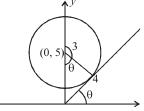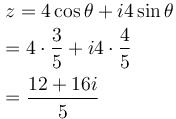*Answer can only contain numeric values
QUESTION: 5

If origin and non-real roots of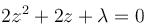form three vertices of an equilateral triangle in argand plane then λ is :

Solution: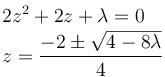for non-real roots 4 - 8λ<0
λ > 1/2
for equilateral triangle
|z1 – z2 | = |z2 – z3 | = |z3 – z1 |
⇒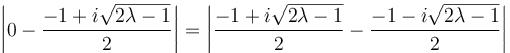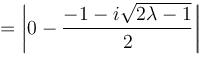or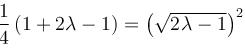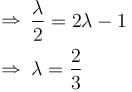*Answer can only contain numeric values
QUESTION: 6

z1 , z2 , z3  are three vertices of an equilateral triangle circumscribing the circle  If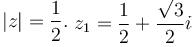and z1 , z2 , z3  are in anticlockwise sense then zis :

Solution: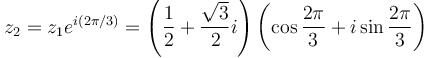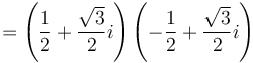= –1

*Answer can only contain numeric values
QUESTION: 7

Equation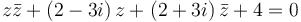represent a circle of radius :

Solution:

Compare with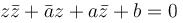a = 2 + 3i, b = 4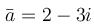So, radius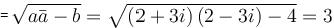*Answer can only contain numeric values
QUESTION: 8

Find the value of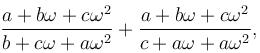here ω is complex cube root of unity.

Solution: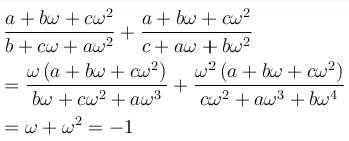*Answer can only contain numeric values
QUESTION: 9

If  iz3 + z2 - z + i = 0,  then |z|  equal to :

Solution:

iz3 + z2 – z + i = 0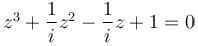z3 – iz2 + iz + 1 = 0
z2 (z – i) + i(z – i) = 0
(z – i)(z2 + i) = 0
(z – i)(z2 + i) = 0
z = i or z2 = –i
|z| = |i|   or    |z|2 = |–i|
⇒ |z| = 1  or  |z|2 = 1
So,  |z| = 1

*Answer can only contain numeric values
QUESTION: 10

If x, y  are real and  –3 + x2 yi, x2 + y + 4i  are conjugate of each other, then |x| + |y| is equal to :

Solution:

–3 – x2 yi = x2+ y + 4i
So,  x2 + y = –3
⇒ y = –3 – x2
x2y = –4
⇒ x2 (3 + x2 ) = 4
x4 + 3x2 – 4 = 0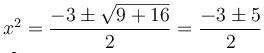x2 = –4, 1 (x is real)
|x| = 1
y = –4   |y| = 4
|x| + |y| = 5